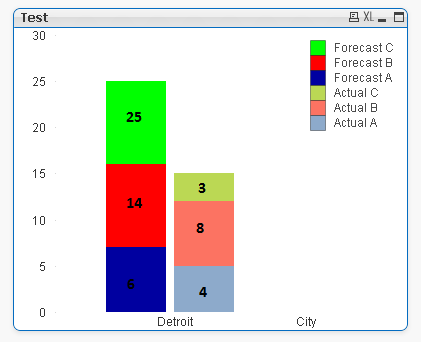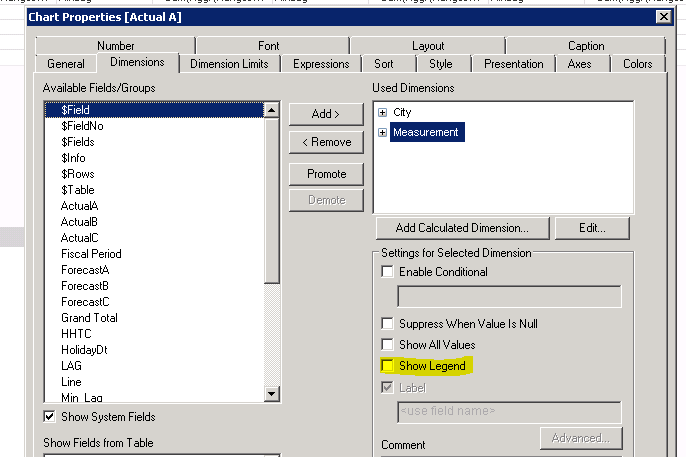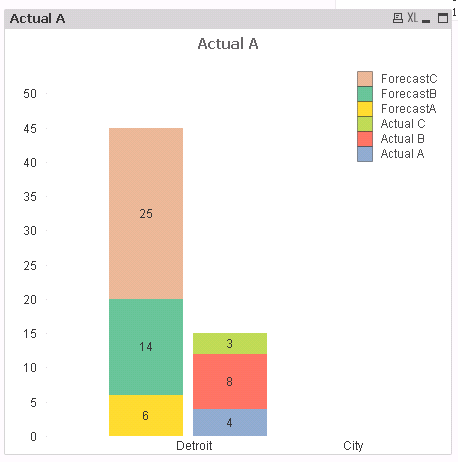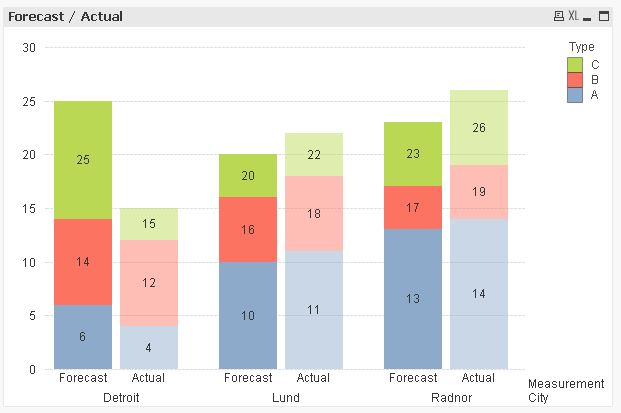# QlikView App Dev

Discussion Board for collaboration related to QlikView App Development.

Announcements
Skip the ticket, Chat with Qlik Support instead for instant assistance.
cancel
Showing results for
Did you mean:Anonymous
Not applicable

## Is there anyway I can replicate this chart in QlikView?

I am struggling to create this chart in Qlik. I can create the bars by expressions like Forecast C = Forecast C- Forecast B- Forecast A. but how can I show the Actual values?6 RepliesChampion III

Can you share your sample file with some data to look into?Anonymous
Not applicable
Author

Actually, I need to create a graph like this so I don't have any qvw file but I can share the script for the bars.

City, ForecastA, ForecastB, ForecastC, Measurement

Detroit, 6, 14, 25, Forecast

];

City, ActualA, ActualB, ActualC, Measurement

Detroit, 4, 12, 15, Actual

];

Forecast bar is what I want to do and Actual bar shows what I can accomplish.Champion III

Try this?

City:

City, ForecastA, ForecastB, ForecastC, Measurement

Detroit, 6, 14, 25, Forecast

];

Concatenate

City, ActualA, ActualB, ActualC, Measurement

Detroit, 4, 12, 15, Actual

];

Dim: like below image

For measurement dimension uncheck Show Legend.= Sum(ActualA)

= Sum(ActualB)-Sum(ActualA)

=Sum(ActualC) -Sum(ActualB)

= Sum(ForecastA)

=Sum(ForecastB)

=Sum(ForecastC)

Below is what you getAnonymous
Not applicable
Author

Yes. I can replicate that but what I want is to 25 bar as 25-14-6 = 5 height, 14 bar as 8 respectively. but the number inside the bar should show 25,14,6. Let me know if you do not understand my question. Thank you.Champion III

try below for Forecast expressions:

= Sum(ForecastA)

= Dual(Sum(ForecastB), Sum(ForecastB) - Sum(ForecastA))

=Dual(Sum(ForecastC), Sum(ForecastC) - Sum(ForecastB) - Sum(ForecastA))MVP

Hi,

maybe one solution could be:Dual(Sum(Value),RangeSum(Sum(Value),-Above(Sum(Value))))Color(RowNo()) bitand ARGB(Pick(Match(Measurement,'Actual','Forecast'),120,255),255,255,255)

```tabValues:
CrossTable (Type, Value, 2)
City, Measurement, A, B, C
Detroit, Forecast, 6, 14, 25
Lund, Forecast, 10, 16, 20
];

CrossTable (Type, Value, 2)
City, Measurement, A, B, C
Detroit, Actual, 4, 12, 15
Lund, Actual, 11, 18, 22